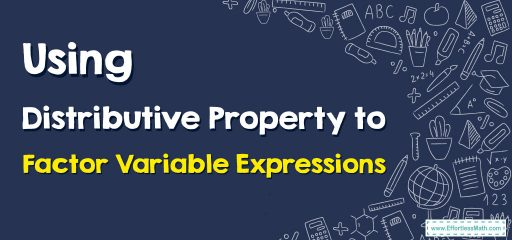# Using Distributive Property to Factor Variable Expressions

In this article, the focus is on teaching you how to Using Distributive Property to Factor Variable Expressions. By understanding how to Use Distributive Property, you can simply Factor Variable Expressions.The distributive property is a mathematical rule that states that multiplying a single value by a sum of values is the same as multiplying each individual value in the sum by that single value and then adding the products.

## A step-by-step guide toidentifying equivalent expressions

The distributive property can also be used to factor in variable expressions.

To factor variable expressions utilizing the distributive property, follow these steps:
Step 1: Multiply or distribute the outer term to the inner terms.
Step 2: Then, combine the like terms.
Step 3: Write terms in a way that the constants and variables are on the opposite sides of the equal’s sign.
Step 4: Finally, resolve the equation and simplify.

### Using Distributive Property to Factor Variable Expressions – Example 1

Factor the expression, $$8p+16$$t. Write a product with a whole number greater than 1.
Solution:
Step 1: Find the greatest common factor of 8p and 16t. It is 8.
Step 2: Divide each number by 8. $$8÷8=1, 16÷8=2$$
Step 3: Use the distributive property to write an equivalent expression.
$$8p+16t=8×p+8×2t=8(p+2t)$$

### Using Distributive Property to Factor Variable Expressions – Example 2

Factor the expression, $$70+30n$$. Write a product with a whole number greater than 1.
Step 1: Find the greatest common factor of 70 and 30n. It is 10.
Step 2: Divide each number by $$10. 70÷10=7, 30÷10=3$$
Step 3: Use the distributive property to write an equivalent expression.
$$70+30n=10×7+10×3n=10(7+3n)$$

### What people say about "Using Distributive Property to Factor Variable Expressions - Effortless Math: We Help Students Learn to LOVE Mathematics"?

No one replied yet.

X
30% OFF

Limited time only!

Save Over 30%

SAVE $5 It was$16.99 now it is \$11.99